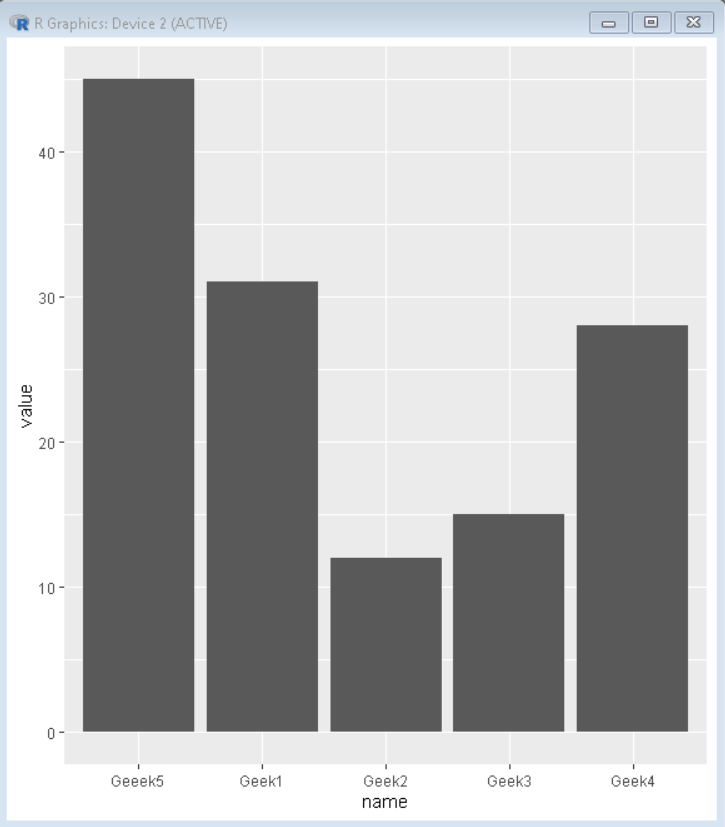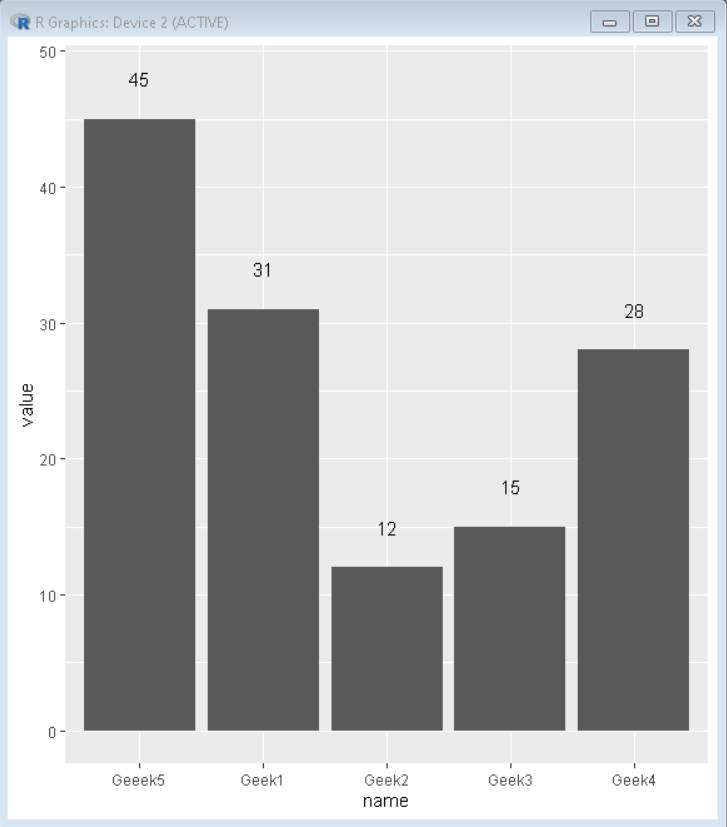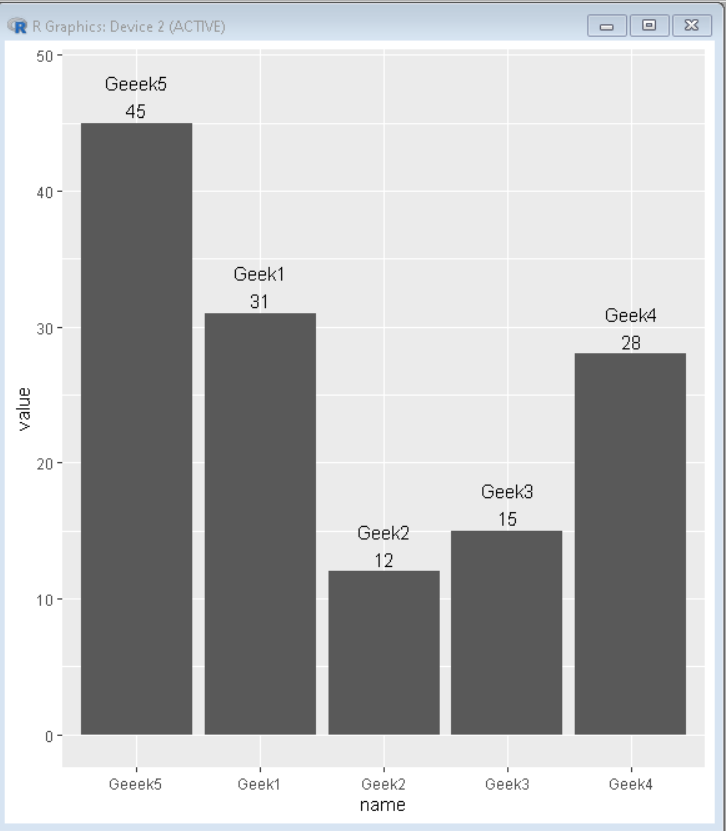# How to Add Labels Over Each Bar in Barplot in R?

• Last Updated : 18 Oct, 2021

In this article, we will see how to add labels over each bar in barplot in R Programming language.

To add labels on top of each bar in Barplot in R we use the geom_text() function of the ggplot2 package.

Syntax: plot+ geom_text(aes(label = value, nudge_y )

Parameters:

• value: value field of which labels have to display.
• nudge_y: distance shift in the vertical direction for the label

### Creating a basic barplot with no labels on top of bars:

In the below example, we will create dataframe and then plot a barplot with this dataframe with no labels.

## R

 `# Create sample data``set.seed``(5642)                            ``sample_data <- ``data.frame``(name = ``c``(``"Geek1"``,``"Geek2"``,``                                   ``"Geek3"``,``"Geek4"``,``                                   ``"Geeek5"``) ,``                          ``value = ``c``(31,12,15,28,45))``# Load ggplot2 package``library``(``"ggplot2"``)` `# Create bar plot``plot<-``ggplot``(sample_data,``             ``aes``(name,value)) +``geom_bar``(stat = ``"identity"``)``plot`

Output:## Get labels on the top of bars

In the below example, we will add geom_text() in the plot to get labels on top of each bar.

## R

 `# Create sample data``set.seed``(5642)                            ``sample_data <- ``data.frame``(name = ``c``(``"Geek1"``,``"Geek2"``,``                                   ``"Geek3"``,``"Geek4"``,``                                   ``"Geeek5"``) ,``                          ``value = ``c``(31,12,15,28,45))``# Load ggplot2 package``library``(``"ggplot2"``)` `# Create bar plot with labels``plot<-``ggplot``(sample_data,``             ``aes``(name,value)) +``geom_bar``(stat = ``"identity"``)+``geom_text``(``aes``(label = ``signif``(value)), nudge_y = 3)``plot`

Output:## Multiple labels on the top of bars

By adjusting the nudge_y values you can add multiple label on top of bars.

## R

 `# Create sample data``set.seed``(5642)                            ``sample_data <- ``data.frame``(name = ``c``(``"Geek1"``,``"Geek2"``,``                                   ``"Geek3"``,``"Geek4"``,``                                   ``"Geeek5"``) ,``                          ``value = ``c``(31,12,15,28,45))``# Load ggplot2 package``library``(``"ggplot2"``)` `# Create bar plot with labels``plot<-``ggplot``(sample_data,``             ``aes``(name,value)) +``geom_bar``(stat = ``"identity"``)+``geom_text``(``aes``(label = ``signif``(value)), nudge_y = 1) +``geom_text``(``aes``(label = name), nudge_y = 3)``plot`

Output:My Personal Notes arrow_drop_up